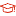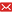UM  > Faculty of Science and Technology  > DEPARTMENT OF MATHEMATICS
 Residential College false Status 已發表Published Exponential-sum-approximation technique for variable-order time-fractional diffusion equations Zhang, Jia Li; Fang, Zhi Wei; Sun, Hai Wei2022-02-01 Source Publication Journal of Applied Mathematics and ComputingISSN 1598-5865 Volume 68Issue:1Pages:323-347 Abstract In this paper, we study the variable-order (VO) time-fractional diffusion equations. For a VO function α(t) ∈ (0 , 1) , we develop an exponential-sum-approximation (ESA) technique to approach the VO Caputo fractional derivative. The ESA technique keeps both the quadrature exponents and the number of exponentials in the summation unchanged at different time level. Approximating parameters are properly selected to achieve the efficient accuracy. Compared with the general direct method, the proposed method reduces the storage requirement from O(n) to O(log n) and the computational cost from O(n) to O(nlog n) , respectively, with n being the number of the time levels. When this fast algorithm is exploited to construct a fast ESA scheme for the VO time-fractional diffusion equations, the computational complexity of the proposed scheme is only of O(mnlog n) with O(mlog n) storage requirement, where m denotes the number of spatial grid points. Theoretically, the unconditional stability and error analysis of the fast ESA scheme are given. The effectiveness of the proposed algorithm is verified by numerical examples. Keyword Exponential-sum-approximation Method Fast Algorithm Stability And Convergence Time-fractional Diffusion Equation Variable-order Caputo Fractional Derivative DOI 10.1007/s12190-021-01528-7 URL View the original Indexed By SCIE Language 英語English WOS Research Area Mathematics WOS Subject Mathematics, Applied ; Mathematics WOS ID WOS:000632851600001 Scopus ID 2-s2.0-85103191249 Fulltext Access Citation statistics Cited Times [WOS]:12   [WOS Record]     [Related Records in WOS] Document Type Journal article Collection DEPARTMENT OF MATHEMATICS Corresponding Author Sun, Hai Wei Affiliation Department of Mathematics, University of Macau, Macao First Author Affilication University of Macau Corresponding Author Affilication University of Macau Recommended CitationGB/T 7714 Zhang, Jia Li,Fang, Zhi Wei,Sun, Hai Wei. Exponential-sum-approximation technique for variable-order time-fractional diffusion equations[J]. Journal of Applied Mathematics and Computing,2022,68(1):323-347. APA Zhang, Jia Li,Fang, Zhi Wei,&Sun, Hai Wei.(2022).Exponential-sum-approximation technique for variable-order time-fractional diffusion equations.Journal of Applied Mathematics and Computing,68(1),323-347. MLA Zhang, Jia Li,et al."Exponential-sum-approximation technique for variable-order time-fractional diffusion equations".Journal of Applied Mathematics and Computing 68.1(2022):323-347.
 Files in This Item: There are no files associated with this item.
 Related Services Recommend this item Bookmark Usage statistics Export to Endnote Google Scholar Similar articles in Google Scholar [Zhang, Jia Li]'s Articles [Fang, Zhi Wei]'s Articles [Sun, Hai Wei]'s Articles Baidu academic Similar articles in Baidu academic [Zhang, Jia Li]'s Articles [Fang, Zhi Wei]'s Articles [Sun, Hai Wei]'s Articles Bing Scholar Similar articles in Bing Scholar [Zhang, Jia Li]'s Articles [Fang, Zhi Wei]'s Articles [Sun, Hai Wei]'s Articles Terms of Use No data! Social Bookmark/Share